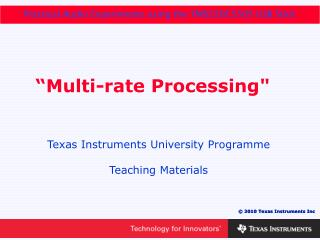# “Multi-rate Processing" - PowerPoint PPT PresentationDownload Presentation“Multi-rate Processing"

“Multi-rate Processing"Download Presentation## “Multi-rate Processing"

- - - - - - - - - - - - - - - - - - - - - - - - - - - E N D - - - - - - - - - - - - - - - - - - - - - - - - - - -
##### Presentation Transcript

1. “Multi-rate Processing" Texas Instruments University Programme Teaching Materials

2. Multi-Rate Processing

3. Introduction • So far we have used fixed sampling rates of 48000 Hz and 8000 Hz • It is possible to change sampling rate inside a digital signal processing (DSP) application. This is known as multi-rate processing • It is a technique that can be used to improve the performance of digital filters.

4. Objectives • To show the effect of sampling frequency on the performance of digital filters • To change sampling rate using “up sampling” and “down sampling” • To introduce fractional digital delays • To implement the “phasing” audio effect on the Texas Instruments TMS320C5505 USB Stick with a microphone/electric guitar and headphones/computer loudspeakers.

5. Effect of Sampling Rate

6. 500 Hz High Pass Filter Fs = 48000 Hz Poor: only -7dB

7. 500 Hz High Pass Filter Fs = 8000 Hz Good: -60 dB

8. Reducing the Sampling Rate • The process of changing from a high sampling rate to a low sampling rate is called “down sampling”. Fs = 48000 Hz Ignore Use only 1 sample in 6 Fs = 8000 Hz

9. Nyquist Frequency • At Fs = 8000 Hz, the highest frequency that can be represented is FN = Fs/2 = 4000 Hz. • Before down sampling, remove all frequencies above FN = 4000 Hz using a low-pass “anti-aliasing” filter.

10. Multi-rate Processing Model Use 1 sample in 6 Anti-aliasing filter

11. Matlab Model Frequency Responses Fs = 48000 Hz Fs = 8000 Hz kHz kHz Poor low-frequency performance Good low-frequency performance

12. Increasing Sampling Rate • The process of converting from a low sampling to a high sampling rate is known as “up sampling” • Three steps are involved: • Fill gaps with zeroes • Apply “anti-imaging” filter to smooth output • Apply gain.

13. Up Sampling Example Fs = 8000 Hz Filter Fill with zeroes 0 0 0 0 0 0 0 0 0 0 Fs = 48000 Hz

14. Multi-rate Filtering

15. Multi-rate Filter Outputs Bass component Treble component kHz kHz Good low-frequency performance

16. Fractional Delays

17. Digital Delays • Some typical digital delays are: • z-1 => 1 sample delay • z-2 => 2 sample delay • z-3 => 3 sample delay • How do we implement: • z-1.5 => 1.5 sample delay?

18. Delays for Different Fs • Suppose Fs = 48000 Hz and delay = 9 samples: • H(z) = z-9 • Now let us “down sample” to Fs = 8000 Hz. Divide • delay by 48000/8000 = 6. • H(z) = z-9/6 = z-1.5 • By “down sampling” we have created a delay of • 1.5 samples.

19. Fractional Delay Model

20. Effect of Delay Samples Delay 1.5 samples Delay 2.5 samples kHz kHz Zero moves position with delay length

21. Phasing

22. Phasing Audio Effect • The “phasing” audio effect uses an automatically changing notch filter to cancel out a range of audio frequencies • Click on the icon below to hear the effect.

23. Implementing Phasing • Some possible ways to implement phasing: • IIR Notch Filters • best using a floating-point processor • Integer Delays • does not sweep all frequencies and leaves gaps • Fractional Delays • small steps • easy to implement • best choice for TMS320C5505 USB Stick.

24. Effect of Different Delays Slowly increase number of delays in fractional steps kHz kHz kHz Zero moves with changing delay length

25. Phasing Block Diagram

26. C Code Implementation

27. C Code Implementation • For multi-rate processing, the FIR filters use the C-callable assembly language functions in FIR_filter_asm.asm • The code for “phasing” can be found in the module phasing.c

28. Phasing Implementation • Uses the TMS320C5505 / TMS320C5515 USB Stick running at Fs = 48000 Hz sampling rate at the codec • Has a 4000 Hz anti-aliasing filter before “down sampling” to Fs = 8000 Hz • Output uses Fs = 8000 Hz.

29. Introduction to Laboratory

30. USB Stick Setup TMS320C5505 USB to PC Microphone Headphones

31. USB Stick Setup TMS320C5505 USB to PC Headphones Electric Guitar

32. Installing the Application • The code is provided in Application 10 Multirate Processing • Follow the steps previously given in Chapter 1 to set up the new project Multirate Processing.

33. Create New CCS Project

34. Files in Project

35. Console

36. Experiments

37. Compare Filter Performance • Compare the effect of the two different methods of filtering: • 2 Flashes = single rate filtering • 3 Flashes = multi-rate filtering.

38. Changing Phasing Effect • Change the LENGTH of the buffer to a value other than 32.

39. Changing the Sweep Rate This variable controls the sweep rate. Try other values.

40. Add to Guitar Effects • Add the phasing effect to the Guitar Effects Application 9.

41. Programming Challenge • Modify the counter to count both up and down:

42. Questions • How does sampling frequency Fs affect the performance of digital filters? • How can fractional digital delays be implemented? • What is an “anti-aliasing” filter? • What is an “anti-imaging filter”?

43. References • Digital Signal Processing, A Practical Approach by Emmanuel C. Ifeachor and Barrie W. Jervis. ISBN 0201-59619-9.